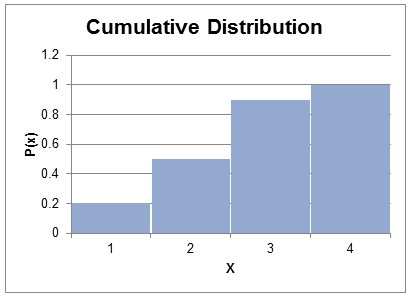# Cumulative Distribution Function

We can also construct a cumulative distribution function for a random variable. A cumulative distribution function gives the probability that the random variable X is less than or equal to x, for every value x. In case of discrete random variables, the cumulative distribution function is the sum of the probabilities of all outcomes unto and including the specific outcome x.

The cumulative distribution function is expressed as  .

We will build upon our earlier probability distribution example.

 xi P(xi) F(xi) 1 0.2 0.2 2 0.3 0.5 3 0.4 0.9 4 0.1 1

Probability that X =1 is 0.2

Probability that X = 1 or 2 = 0.2 + 0.3 = 0.5

Probability that X = 1 or 2 or 3 = 0.2 + 0.3 +0.4 = 0.9

Probability that X = 1 or 2 or 3 or 4= 0.2 + 0.3 +0.4 +0.1= 1.0

The histogram for cumulative distribution will look as follows:The above cumulative distribution was for a discrete random variable. Even a continuous random variable will have a cumulative distribution function.